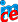# An ECCOMAS Advanced Course on Computational Structural Dynamics

## The program of the short course

#### Monday

1. Basics of dynamics (M. Okrouhlík)
- Historical background
- Newton’s laws
- Newtonian, Lagrangian and Hamiltonian mechanics
2. Continuum mechanics I (J. Plešek)
- Kinematics of deformation
- Strains and stresses
- Governing equations
3. Continuum mechanics II (J. Plešek)
- Convexity condition
- Basics of thermodynamics
- Constitutive equations for small and large strains
4. Continuum mechanics III (R. Ohayon)
- Variational formulations in linear dynamics and vibrations
- Modal analysis
- Rayleigh quotient, Hamilton’s principle
5. Dynamics of multibody systems (A. Ibrahimbegovic)
- Governing equations
- Constrains
- Lagrange equations and Lagrange multipliers
- Numerical methods in multibody dynamics

#### Tuesday

6. Finite element method I (J. González)
- Principle of virtual work
- Finite Element Formulation
- Assembly of global matrices
- Convergence properties
- Examples
7. Finite element method II (A. Ibrahimbegovic)
- Shape functions and higher order FEM
- Isoparametric formulation
- Numerical integration
- Hybrid and mixed formulation, inf-sup condition
8. Finite element method III (A. Tkachuk)
- Locking phenomena and hourglass effect
- Assumed strain, enhanced strain FEM, B-bar formulation
- Reduced integration and stabilization
9. Finite element method IV (J. Kruis)
- Linear solvers in FEM
- Matrix factorization
- Sparse solvers, Krylov methods (especially conjugate gradient method)
10. Finite element method V (A. Ibrahimbegovic)
- FEM for nonlinear problems
- Solvers for nonlinear static problems - NR, BGFS, semi-Newton methods, etc.
- Convergence criteria

#### Wednesday

11. Finite element method VI (J. Kruis)
- FEM in vibration problems, mass matrix
- Spectral and modal analysis
- Numerical methods for eigen-value problem (subspace iteration, etc)
- Convergence of FEM in eigen-value problem
12. Direct time integration in dynamics I (R. Kolman)
- FEM in linear dynamics, formulation of dynamic problems
- Introduction into direct time integration
- Basic methods (Newmark method and central difference method)
- Lumping techniques for mass matricesStability of time schemes
- Stability of time schemes
13. Finite element method VII (A. Ibrahimbegovic)
- Dynamic problems
- Solving of nonlinear time-depend problems
14. Finite element method VIII (A. Combescure)
- Basics of shell theory
- FEM shell models
- FEM for shells in dynamics
- Mass matrices for shells
15. Direct time integration in dynamics II (A. Tkachuk)
- Time step size estimates – global/local estimate in FEM
- Treatment of time step size – mass scaling, bi-penalty, etc
- Application in crash problems

#### Thursday

16. Modal reduction and reduction methods in dynamics (R. Ohayon)
- Variational analysis of dynamic sub-structuring
- Substructuring analysis in discretized (finite element) case
- Hurty and Craig-Bampton methods
17. Partitioned analysis I – basic theory (K.C. Park)
- Theory of Lagrange multipliers
- Basic theory of partitioned analysis
- Equations of motion for partitioned systems
18. Partitioned analysis II (K.C. Park)
- Domain decomposition methods
- Finite element tearing and interconnect (FETI)
- Coupling of FEM/FEM
19. Dynamic contact problems (A. Tkachuk)
- FEM in contact problems
- Penalty method
- Augmented Lagrangian method
- Mortar methods
20. Finite element method - wave propagation (R. Kolman)
- Theory of wave propagation in elastic solids
- Wave speeds in solids
- Dispersion and frequency analysis of FEM
- Numerical benchmarks

#### Friday

21. Modern methods of direct time integration (A. Combescure)
- Generalized time schemes
- Asynchronous and variational schemes
- Sub-cycling methods, coupling of different time schemes
22. Coupled problems – Fluid-structures interactions (K.C. Park)
- Variational  formulation
- Methods of discretizations
- Staggered analysis
23. Boundary element method (J. González)
- Introduction into Boundary Element Method
- Linear Acoustics
- Coupling FEM/BEM
24. Numerical methods for dynamic crack propagation (A. Combescure)
- Extended finite element method (XFEM)
- Meshless methods and Level-set methods
- Cohesive FEM
25. Vibroacoustic/elastoacoustic modelling (R. Ohayon)
- General local equations of vibroacoustic problem
- Choice of unknown fields and variational formulations of the problem
- Finite element discretization and Reduced Order Models for the interior problemPowered by Imce 3.01  © 2015, Pavel Formánek, Institute of Thermomechanics AS CR, v.v.i. [generated: 0.0351s]